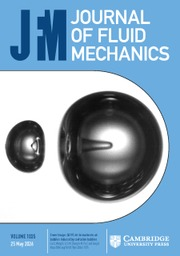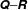Home
Hostname: page-component-558cb97cc8-vrcgq Total loading time: 0.363 Render date: 2022-10-07T11:06:34.224Z Has data issue: true Feature Flags: { "shouldUseShareProductTool": true, "shouldUseHypothesis": true, "isUnsiloEnabled": true, "useRatesEcommerce": false, "displayNetworkTab": true, "displayNetworkMapGraph": false, "useSa": true } hasContentIssue trueJournal of Fluid Mechanics

## Abstract

The velocity gradient tensor for turbulent flow contains crucial information on the topology of turbulence, vortex stretching and the dissipation of energy. A Schur decomposition of the velocity gradient tensor (VGT) is introduced to supplement the standard decomposition into rotation and strain tensors. Thus, the normal parts of the tensor (represented by the eigenvalues) are separated explicitly from non-normality. Using a direct numerical simulation of homogeneous isotropic turbulence, it is shown that the norm of the non-normal part of the tensor is of a similar magnitude to the normal part. It is common to examine the second and third invariants of the characteristic equation of the tensor simultaneously (the$\unicode[STIX]{x1D64C}{-}\unicode[STIX]{x1D64D}$ diagram). With the Schur approach, the discriminant function separating real and complex eigenvalues of the VGT has an explicit form in terms of strain and enstrophy: where eigenvalues are all real, enstrophy arises from the non-normal term only. Re-deriving the evolution equations for enstrophy and total strain highlights the production of non-normality and interaction production (normal straining of non-normality). These cancel when considering the evolution of the VGT in terms of its eigenvalues but are important for the full dynamics. Their properties as a function of location in$\unicode[STIX]{x1D64C}{-}\unicode[STIX]{x1D64D}$ space are characterized. The Schur framework is then used to explain two properties of the VGT: the preference to form disc-like rather than rod-like flow structures, and the vorticity vector and strain alignments. In both cases, non-normality is critical for explaining behaviour in vortical regions.

## JFM classification

Type
JFM Papers
Information
Journal of Fluid Mechanics , 10 August 2018 , pp. 876 - 905
© 2018 Cambridge University Press

## Access options

Get access to the full version of this content by using one of the access options below. (Log in options will check for institutional or personal access. Content may require purchase if you do not have access.)

## References

Ashurst, W. T., Kerstein, A. R., Kerr, R. A. & Gibson, C. H. 1987 Alignment of vorticity and scalar gradient with strain rate in simulated Navier–Stokes turbulence. Phys. Fluids 30, 23432353.CrossRefGoogle Scholar
Betchov, R. 1956 An inequality concerning the production of vorticity in isotropic turbulence. J. Fluid Mech. 1, 497504.CrossRefGoogle Scholar
Biferale, L., Chevillard, L., Meneveau, C. & Toschi, F. 2007 Multiscale model of gradient evolution in turbulent flows. Phys. Rev. Lett. 98, 214501.CrossRefGoogle ScholarPubMed
Cantwell, B. J. 1992 Exact solution of a restricted Euler equation for the velocity gradient tensor. Phys. Fluids A 4 (4), 782793.CrossRefGoogle Scholar
Cantwell, B. J. 1993 On the behavior of velocity gradient tensor invariants in direct numerical simulations of turbulence. Phys. Fluids A 5, 20082013.CrossRefGoogle Scholar
Chong, M. S., Perry, A. E. & Cantwell, B. J. 1990 A general classification of three-dimensional flow fields. Phys. Fluids A 2, 765777.CrossRefGoogle Scholar
Dubief, Y. & Delcayre, F. 2000 On coherent-vortex identification in turbulence. J. Turbul. 1, N11.CrossRefGoogle Scholar
Eberlein, P. J. 1965 On measures of non-normality for matrices. Am. Math. Mon. 72, 995996.CrossRefGoogle Scholar
Elsinga, G. E. & Marusic, I. 2010 Evolution and lifetimes of flow topology in a turbulent boundary layer. Phys. Fluids 22, 015102.CrossRefGoogle Scholar
Girimaji, S. S. & Pope, S. B. 1990 A diffusion model for velocity gradients in turbulence. Phys. Fluids 2 (2), 242256.CrossRefGoogle Scholar
Golub, G. H. & van Loan, C. F. 2013 Matrix Computations, 4th edn. Johns Hopkins University Press.Google Scholar
Goto, S. 2008 A physical mechanism of the energy cascade in homogeneous isotropic turbulence. J. Fluid Mech. 605, 355366.CrossRefGoogle Scholar
Henrici, P. 1962 Bounds for iterates, inverses, spectral variation and fields of values of non-normal matrices. Numer. Math. 4, 2440.CrossRefGoogle Scholar
Higham, J. E., Brevis, W. & Keylock, C. J. 2016 A rapid non-iterative proper orthogonal decomposition based outlier detection and correction for PIV data. Meas. Sci. Technol. 27, 125303.CrossRefGoogle Scholar
Hunt, J. C. R., Wray, A. A. & Moin, P.1988 Eddies, stream, and convergence zones in turbulent flows. Tech. Rep. CTR-S88. Center for Turbulence Research, Stanford University.Google Scholar
Jeong, E. & Girimaji, S. S. 2003 Velocity-gradient dynamics in turbulence: effect of viscosity and forcing. Theor. Comput. Fluid Dyn. 16, 421432.CrossRefGoogle Scholar
Jimenez, J. 1992 Kinematic alignmernt effects in turbulent flows. Phys. Fluids A 4, 652654.CrossRefGoogle Scholar
Johnson, P. L. & Meneveau, C. 2016 A closure for Lagrangian velocity gradient evolution in turbulence using recent-deformation mapping of initially Gaussian fields. J. Fluid Mech. 804, 387419.CrossRefGoogle Scholar
Kerr, R. M. 1985 Higher-order derivative correlations and the alignment of small-scale structures in isotropic, numerical turbulence. J. Fluid Mech. 153, 3158.CrossRefGoogle Scholar
Keylock, C. J. 2017 Synthetic velocity gradient tensors and the identification of statistically significant aspects of the structure of turbulence. Phys. Rev. Fluids 2, 084607.CrossRefGoogle Scholar
Kuo, A. Y.-S. & Corrsin, S. 1972 Experiment on the geometry of the fine-structure regions in fully turbulent fluid. J. Fluid Mech. 56, 447479.CrossRefGoogle Scholar
Lawson, J. M. & Dawson, J. R. 2015 On velocity gradient dynamics and turbulent structure. J. Fluid Mech. 780, 6098.CrossRefGoogle Scholar
Lee, S. L. 1995 A practical upper bound for departure from normality. SIAM J. Matrix Anal. Applics. 16, 462468.CrossRefGoogle Scholar
Li, Y. & Meneveau, C. 2007 Material deformation in a restricted Euler model for turbulent flows: analytic solution and numerical tests. Phys. Fluids 19, 015104.CrossRefGoogle Scholar
Li, Y., Perlman, E., Wan, M., Yang, Y., Burns, R., Meneveau, C., Chen, S., Szalay, A. & Eyink, G. 2008 A public turbulence database cluster and applications to study Lagrangian evolution of velocity increments in turbulence. J. Turbul. 9, N31.CrossRefGoogle Scholar
Lund, T. S. & Rogers, M. M. 1994 An improved measure of strain state probability in turbulent flows. Phys. Fluids 6 (5), 18381847.CrossRefGoogle Scholar
Lundgren, T. S. 1982 Strained spiral vortex model for turbulent structures. Phys. Fluids 25, 21932203.CrossRefGoogle Scholar
Lüthi, B., Holzner, M. & Tsinober, A. 2009 Expanding the [[()[]mml:mi[]()]]𝙌[[()[]/mml:mi[]()]]–[[()[]mml:mi[]()]]𝙍[[()[]/mml:mi[]()]] space to three dimensions. J. Fluid Mech. 641, 497507.CrossRefGoogle Scholar
Martin, J., Dopazo, C. & Valiño, L. 1998 Dynamics of velocity gradient invariants in turbulence: restricted Euler and linear diffusion models. Phys. Fluids 10, 20122025.CrossRefGoogle Scholar
Meneveau, C. 2011 Lagrangian dynamics and models of the velocity gradient tensor in turbulent flows. Annu. Rev. Fluid Mech. 43, 219245.CrossRefGoogle Scholar
Nomura, K. K. & Post, G. K. 1998 The structure and dynamics of vorticity and rate of strain in incompressible homogeneous turbulence. J. Fluid Mech. 377, 6597.CrossRefGoogle Scholar
Ohkitani, K. & Kishiba, S. 1995 Nonlocal nature of vortex stretching in an inviscid fluid. Phys. Fluids 7 (2), 411421.CrossRefGoogle Scholar
Paul, I., Papadakis, G. & Vassilicos, J. C. 2017 Genesis and evolution of velocity gradients in a spatially developing turbulence. J. Fluid Mech. 815, 295332.CrossRefGoogle Scholar
Perry, A. E. & Chong, M. S. 1987 Description of eddying motions and flow patterns using critical-point concepts. Annu. Rev. Fluid Mech. 19, 125155.CrossRefGoogle Scholar
Reddy, S. C., Schmid, P. J. & Henningson, D. S. 1993 Pseudospectra of the Orr-Sommerfeld operator. SIAM J. Appl. Maths 53, 1547.CrossRefGoogle Scholar
Schur, I. 1909 Über die charakteristischen Wurzeln einer linearen Substitution mit einer Anwendung auf die Theorie der Integralgleichungen. Math. Ann. 66, 488510.CrossRefGoogle Scholar
Taylor, G. I. 1938 The spectrum of turbulence. Proc. R. Soc. Lond. A 164, 476490.CrossRefGoogle Scholar
Trefethen, L. N. & Embree, M. 2005 Spectra and Pseudospectra: The Behavior of Nonnormal Matrices and Operators. Princeton University Press.Google Scholar
Trefethen, L. N., Trefethen, A. E., Reddy, S. C. & Driscoll, T. A. 1993 Hydrodynamic stability without eigenvalues. Science 261, 578584.CrossRefGoogle ScholarPubMed
Tsinober, A. 2001 Vortex stretching versus production of strain/dissipation. In Turbulence Structure and Vortex Dynamics (ed. Hunt, J. C. R. & Vassilicos, J. C.), pp. 164191. Cambridge University Press.Google Scholar
Tsinober, A., Shtilman, L. & Vaisburd, H. 1997 A study of properties of vortex stretching and enstrophy generation in numerical and laboratory turbulence. Fluid Dyn. Res. 21, 477494.CrossRefGoogle Scholar
Vieillefosse, P. 1984 Internal motion of a small element of fluid in an inviscid flow. Physica A 125, 150162.CrossRefGoogle Scholar
Wan, M., Chen, S., Eyink, G., Meneveau, C., Perlman, E., Burns, R., Li, Y., Szalay, A. & Hamilton, S.2016 Johns Hopkins Turbulence Database (JHTDB). http://turbulence.pha.jhu.edu/datasets.aspx.Google Scholar
Wan, M., Xiao, Z., Meneveau, C., Eyink, G. L. & Chen, S. 2010 Dissipation-energy flux correlations as evidence for the Lagrangian energy cascade in turbulence. Phys. Fluids 22 (6), 14.CrossRefGoogle Scholar
Wilczek, M. & Meneveau, C. 2014 Pressure Hessian and viscous contributions to velocity gradient statistics based on Gaussian random fields. J. Fluid Mech. 756, 191225.CrossRefGoogle Scholar
17
Cited by

# Save article to Kindle

To save this article to your Kindle, first ensure coreplatform@cambridge.org is added to your Approved Personal Document E-mail List under your Personal Document Settings on the Manage Your Content and Devices page of your Amazon account. Then enter the ‘name’ part of your Kindle email address below. Find out more about saving to your Kindle.

Note you can select to save to either the @free.kindle.com or @kindle.com variations. ‘@free.kindle.com’ emails are free but can only be saved to your device when it is connected to wi-fi. ‘@kindle.com’ emails can be delivered even when you are not connected to wi-fi, but note that service fees apply.

Find out more about the Kindle Personal Document Service.

The Schur decomposition of the velocity gradient tensor for turbulent flows
Available formats
×

# Save article to Dropbox

To save this article to your Dropbox account, please select one or more formats and confirm that you agree to abide by our usage policies. If this is the first time you used this feature, you will be asked to authorise Cambridge Core to connect with your Dropbox account. Find out more about saving content to Dropbox.

The Schur decomposition of the velocity gradient tensor for turbulent flows
Available formats
×

# Save article to Google Drive

To save this article to your Google Drive account, please select one or more formats and confirm that you agree to abide by our usage policies. If this is the first time you used this feature, you will be asked to authorise Cambridge Core to connect with your Google Drive account. Find out more about saving content to Google Drive.

The Schur decomposition of the velocity gradient tensor for turbulent flows
Available formats
×
×